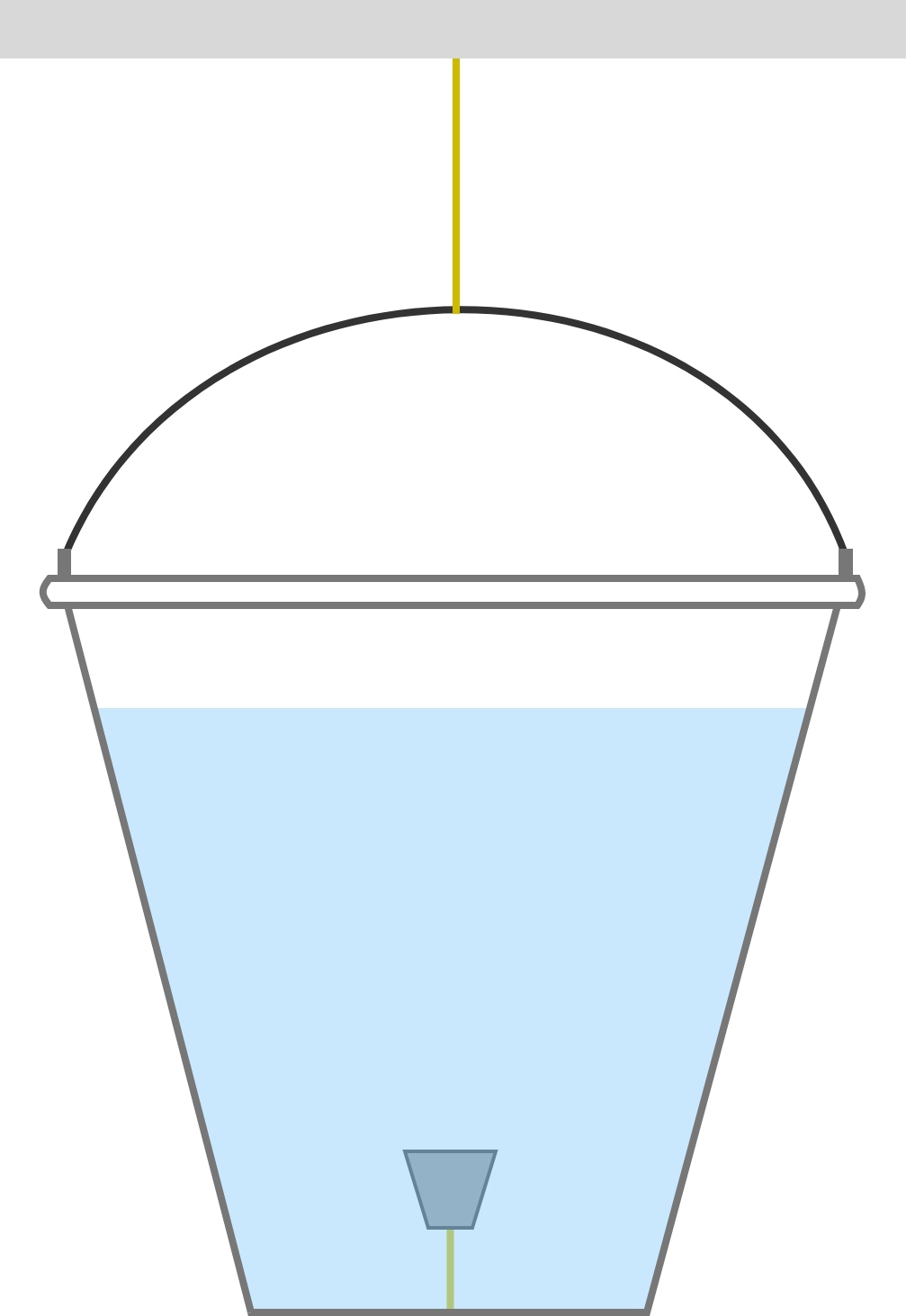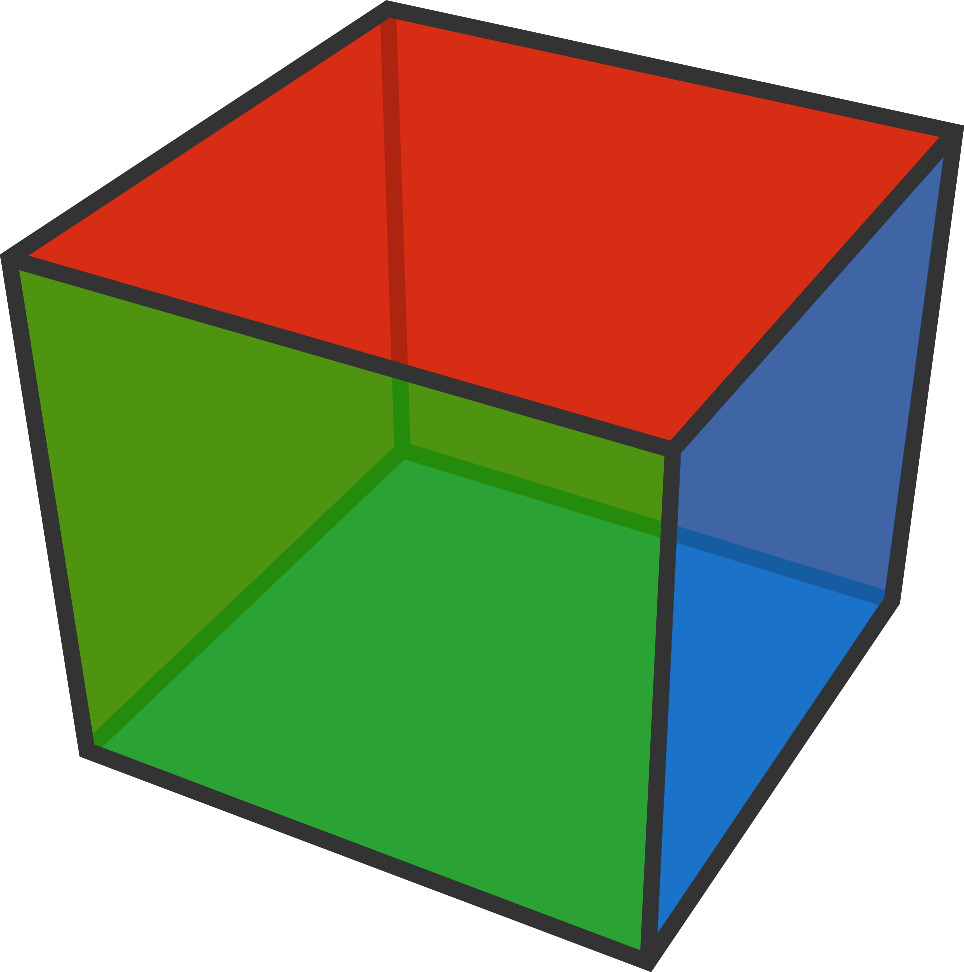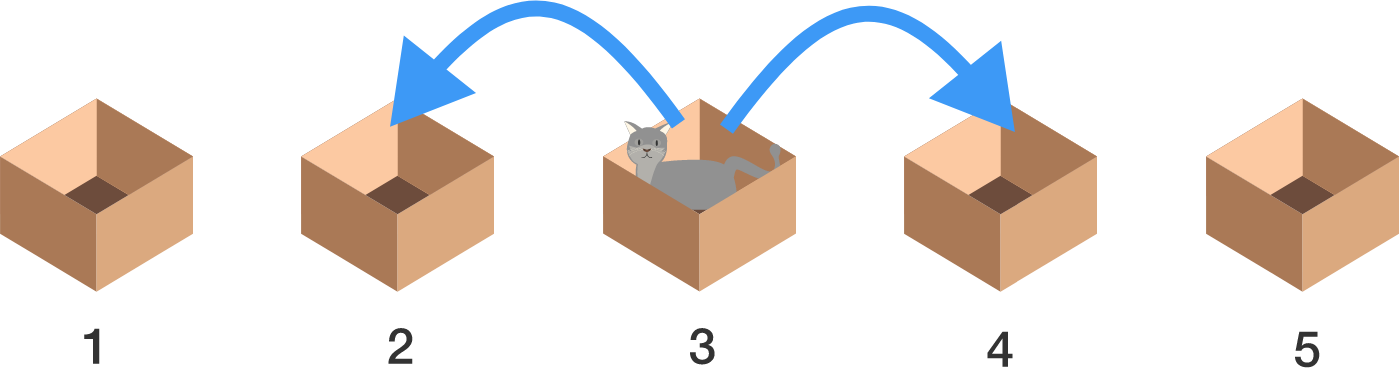# Problems of the Week

Contribute a problem

# 2018-02-19 IntermediateThe diagram shows a bucket filled with water hanging on a string, with a cork tied to the base of the bucket by another string.

When the cork's string is cut, since the cork is less dense than water, the cork will rise up due to buoyancy.

When the string holding the bucket is cut, the bucket and its contents will fall due to gravity.

What happens if both strings are cut simultaneously? How would the cork move relative to the bucket?

How many distinct ways can a cube have $\color{#20A900} \text{1 green face}$, $\color{#3D99F6} \text{2 blue faces}$ and $\color{#D61F06} \text{3 red faces}$?Note: Two ways are only distinct if one cube can't be rotated to look like the other.

There exists an expression with infinite nested roots that evaluates to an integer:

$3=\sqrt{6+\sqrt{6+\sqrt{6+\sqrt{6+\sqrt{6+\cdots}}}}}.$

How many integer values of $x$ between 1 and 1000 (inclusive) are there such that $\sqrt{x+\sqrt{x+\sqrt{x+\sqrt{x+\sqrt{x+\cdots}}}}}$ is also an integer?

A cat hides in one of the 5 boxes labeled 1~5 from left to right, and you need to catch the cat.

Every night it switches places to an adjacent box; for example, if the cat was in #3 and didn't get caught during the day, it moves to #2 or to #4 in the night. Then, everyday when the night is over, you can check only 1 box to find the cat.

Applying the optimal strategy, you are guaranteed to find the cat in at most $X$ days. What is $X?$Note: If the cat was in #5 during the day, it can only move to #4 in the night. Similarly, #1 has only one adjacent box, #2. The boxes are kept closed at all times, but the cat can slip through the folds on top during the night.

$P$ and $Q$ are two distinct, non-constant polynomial functions such that $(P\circ Q)(x) = P(x)Q(x)$ and $P(1) = P(-1) = 2018.$

Find $(Q\circ P)(-1).$

Note: Function composition $(P\circ Q)(x)$ is sometimes denoted as $P\big(Q(x)\big).$

×